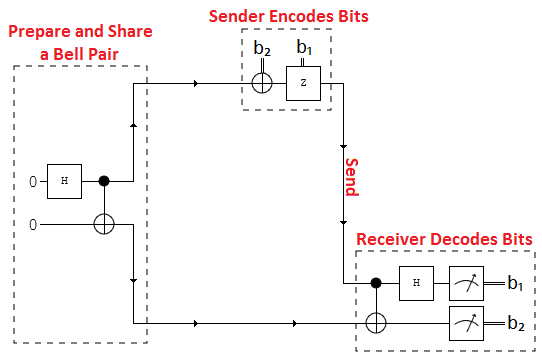### Learn back-end development by writing real code

Boot.dev Blog » Misc » Quantum Programming 101: Superdense Coding Tutorial# Quantum Programming 101: Superdense Coding Tutorial

By Macauley Coggins on Apr 20, 2020

Curated backend podcasts, videos and articles. All free.

If you're looking to become a backend developer, or just stay up-to-date with the latest backend technologies and trends, you found the right place. Subscribe below to get a copy of our newsletter, The Boot.dev Beat, each month in your inbox. No spam, no sponsors, totally free.

## What is Superdense coding?🔗

Superdense coding is a quantum communications protocol that allows a user to send 2 classical bits by sending only 1 qubit.

## The Protocol🔗## Step 1: Preparing the Bell Pair🔗

First a bell pair consisting of 2 qubits is prepared. Where q0 is the senders qubit and q1 is the receivers qubit. To do this q0 is put in to a superposition of states using a hadamard gate.

Then a CNOT operation is performed with q0 being the control and q1 being the target.

## Step 2: Encode The Information On To Q0🔗

Next the sender has to encode the information they want to send on to q0 by applying certain operations to it.

• If they want to send 00 then they perform no operation.
• If they want to send 01 then they perform a Pauli-X operation where q1s state is flipped.
• If they want to send 10 then they apply a Pauli-Z gate.
• If they want to send 11 then apply a Pauli-Z gate followed by a Pauli-X gate

## Step 3: Receiver Decodes the Information🔗

Next q0 is sent and the receiver has to decode the qubit. This is done by applying a CNOT where the received q0 is the control and q1 is the target. Then a hadamard gate is applied to q0.

## How To Run The Program🔗

1. Copy and paste the code below in to a python file
2. Enter your API token in the IBMQ.enable_account(‘Insert API token here’) part
3. Save and run

## Code🔗

``````print('\n Superdense Coding')
print('--------------------------\n')

from qiskit import QuantumRegister, ClassicalRegister, QuantumCircuit, execute,IBMQ

IBMQ.enable_account('INSERT TOKEN HERE')
provider = IBMQ.get_provider(hub='ibm-q')

q = QuantumRegister(2,'q')
c = ClassicalRegister(2,'c')

backend = provider.get_backend('ibmq_qasm_simulator')
print('Provider: ',backend)

#################### 00 ###########################
circuit = QuantumCircuit(q,c)

circuit.h(q) # Hadamard gate applied to q0
circuit.cx(q,q) # CNOT gate applied
circuit.cx(q,q)
circuit.h(q)

circuit.measure(q,c) # Qubits measured

job = execute(circuit, backend, shots=10)

print('Executing Job...\n')
result = job.result()
counts = result.get_counts(circuit)

print('RESULT: ',counts,'\n')

#################### 01 ###########################
circuit = QuantumCircuit(q,c)

circuit.h(q)
circuit.cx(q,q)
circuit.x(q) # X-gate applied
circuit.cx(q,q)
circuit.h(q)

circuit.measure(q,c)

job = execute(circuit, backend, shots=10)

print('Executing Job...\n')
result = job.result()
counts = result.get_counts(circuit)

print('RESULT: ',counts,'\n')

#################### 10 ###########################
circuit = QuantumCircuit(q,c)

circuit.h(q)
circuit.cx(q,q)
circuit.z(q) # Z-gate applied to q0
circuit.cx(q,q)
circuit.h(q)

circuit.measure(q,c)

job = execute(circuit, backend, shots=10)

print('Executing Job...\n')
result = job.result()
counts = result.get_counts(circuit)

print('RESULT: ',counts,'\n')

#################### 11 ###########################
circuit = QuantumCircuit(q,c)

circuit.h(q)
circuit.cx(q,q)
circuit.z(q) # Z-gate applied
circuit.x(q) # X-gate applied
circuit.cx(q,q)
circuit.h(q)

circuit.measure(q,c)

job = execute(circuit, backend, shots=10)

print('Executing Job...\n')
result = job.result()
counts = result.get_counts(circuit)

print('RESULT: ',counts,'\n')
print('Press any key to close')
input()
``````

## Output🔗

After running the code you will see something like the following printed on the screen :InteresteWant to learn about Quantum Programming? Head over to Quantum Computing UK.

For news on Quantum Computing head over to The Quantum Daily